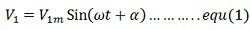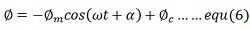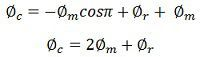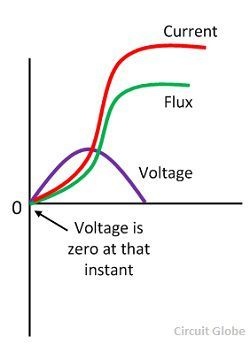# Transformer Inrush Current

Definition: The transformer inrush current is the maximum instantaneous current drawn by the primary of the transformer when their secondary is open circuit. The inrush current does not create any permanent fault, but it causes an unwanted switching in the circuit breaker of the transformer. During the inrush current, the maximum value attained by the flux is over twice the normal flux.

Let a sinusoidal voltageV1 be applied to a transformer, the secondary of which is an open circuit. Here α the angle of the voltage sinusoid at t = 0. Suppose the core loss and primary resistance be neglected, thenWhere T1 is the number of turns and Φ is the flux in the core. In the steady stateFrom equation (1) and equation (2), we get,Integration of the equation (5) givesWhere Φc is the constant or integration to be found from an initial condition at t = 0. Considered that when the transformer is last disconnected from the supply line, a small residual flux Φr remained in the core. Thus, at t = 0, Φ = Φr.

Substituting this value in equation (6) we getThe equation (8) shows that the flux consists of two components, the steady state component Φss and the transient component Φc. The magnitude of the transient componentΦc is a function of α, where α is the instant at which the transformer is switched on to the supply.If the transformer is switched on at α = 0, then cosα = 1.At ωt = π,Thus the core flux attains the maximum value of flux equal to (2φmr) which is over twice the normal flux. This is known as double effecting. Due to this double effect, the core goes into deep saturation. The magnetising current required for producing such a large flux in the core may be as large as ten times the normal magnetising current.Sometimes the RMS value of magnetising current is larger than the primary rated current of the transformer. This current may produce an electromagnetic force which is about twenty-five times the normal value. Therefore the winding of the transformer is strongly braced. The improper operation of protective devices like unwarranted tripping of relays, momentary large voltage drops and large humming due to magnetostriction of the core.

To obtain no transient inrush current, Φc should be zero.

In other words, if the transformer is connected to the supply line near a positive or negative maximum voltage, the inrush current will be minimised. But usually, it is impractical to connect a transformer at a predetermined time in the voltage cycle